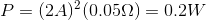# AP Physics C Electricity : Calculating Circuit Power

## Example Questions

### Example Question #19 : Electric Circuits

A straight copper wire has a fixed voltage applied across its length. Which of the following changes would increase the power dissipated by this wire?

None of these options result in increased power dissipation

Decreasing the cross-sectional area of the wire

Increasing the temperature of the wire

Decreasing the length of the wire

Changing the material of the wire to a metal of greater resistivity

Decreasing the length of the wire

Explanation:

Relevant equations: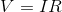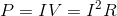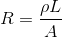Current and resistance are inversely proportional to one another, assuming voltage is fixed. Since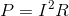, changes in current effect the power more than changes in resistance do. Thus, we need current to increase, meaning that resistance must decrease.

To decrease resistance, we could:

1. Change the material of the wire to one of lesser resistivity

2. Decrease the length of the wire

3. Increase the cross-sectional area of the wire

4. Decrease the temperature of the wire (very slight effect on resistance)

### Example Question #21 : Electric Circuits

A battery is measured to have a potential of 5V. When connected to a wire with no resistors or other components, the voltage measured is 4.9V.

If the current through the wire is measured to be 2A, how much thermal energy is being lost per second as soon as the wire is connected to the battery?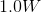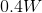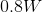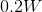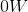Explanation:

First, we must know that the wire has some internal resistance. To calculate this, we need to know the potential drop through the wire, which must be the difference we saw from the initial voltage reading to the second. This value, 0.1V, we plug into Ohm's law to calculate the resistance of the wire.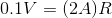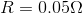The question asks for energy lost per second; this value is equivalent to the power.Use our values to solve.